# Algebra 1 : How to plot fractions on a number line

## Example Questions

### Example Question #1 : How To Plot Fractions On A Number Line

Plot the fraction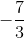on the number line.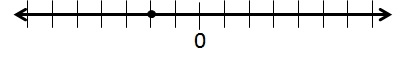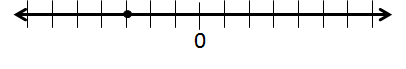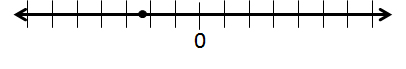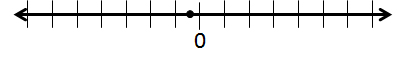The fractionis less thanand greater than, so it must fall between those points on the number line. Negative numbers are to the left ofwhile positive numbers are to the right.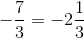Becauseis less than, the point must be closer tothan.